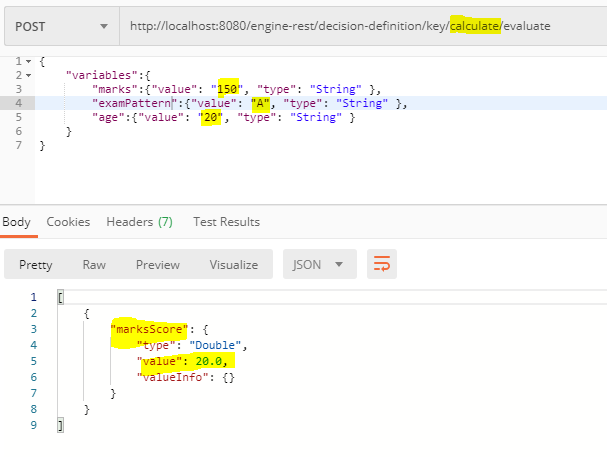# DMN - How to INCLUDE MULTIPLE OUTPUT VARIABLES IN OUTPUT COLUMN

Hello,
I’m implementing DMN in modeler.

(1)It has INPUT PAYLOAD as attached in image (DMN_REQUEST.png).
(2)Diagram is attached in image (DMN_DIAGRAM.png)
(3)Source code file as attached (scoringJames.dmn)

There are 4 output variables and want to send all 4 variables as output variables.
(1)marksScore (2)examPatternScore (3)ageScore (4)total

NOTE:
“(4)total” is calculated by this formula ==> (marksScore
0.2)+(examPatternScore
0.3)+(ageScore
0.2)

Kindly let me know where i’m going wrong or what is to be donescoringJames.dmn (7.2 KB)

Hi @james1980,

thanks for sharing.

I see that you changed the expression language everywhere to `javascript`.

This could be the problem. I think that the expression `"A"` in the “Exam Pattern” input can not be evaluated to `true` because it doesn’t compare the value with the input.

Best regards,
Philipp

@Philipp_Ossler
Thanks,Above is working,But facing issue on calculating “total”

There are 4 output variables and want to send all 4 variables as output variables.
(1)marksScore (2)examPatternScore (3)ageScore (4)total

NOTE:
“(4)total” is calculated by this formula ==> (marksScore
0.2)+(examPatternScore
0.3)+(ageScore
0.2)

Kindly see attached images and let me know what is wrong

The expression can not be evaluate because the variables doesn’t exist. It is not possible to reference a variable from a different output.

You should think about your decision table again. I see the following problems:

• `ageScore` is declared as a string
• `totalScore` is declared as a string
• `totalScope` is an aggregate of the other 3 outputs
• it can not be calculated in this table itself
• maybe not all outputs have a value

An option is to calculate `totalScope` outside of the decision table after it is evaluated.

1 Like

In my opinion the best sokutiin would be a requirements graph. The output variables habe to be double or integer. Thab you define a dependent table and in the output of this table you can repeat the output columns and provide a Fürther output column with the calculation.

Kind regards
David

@davidibl above is working after changing datatype suggested by @Philipp_Ossler .
3 output variables are passed on to script task and “totalscore” is calculated there…
Thanks guys…